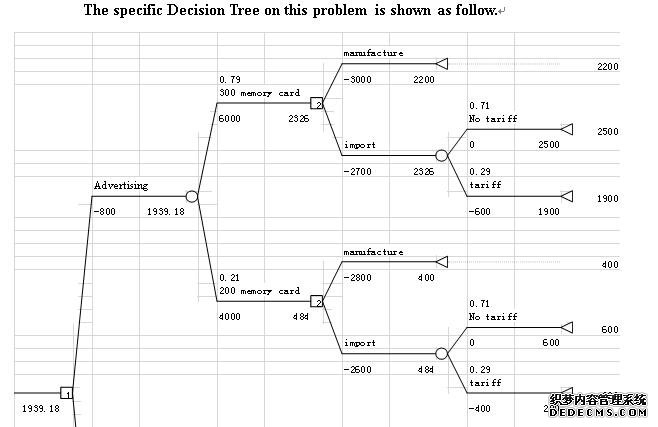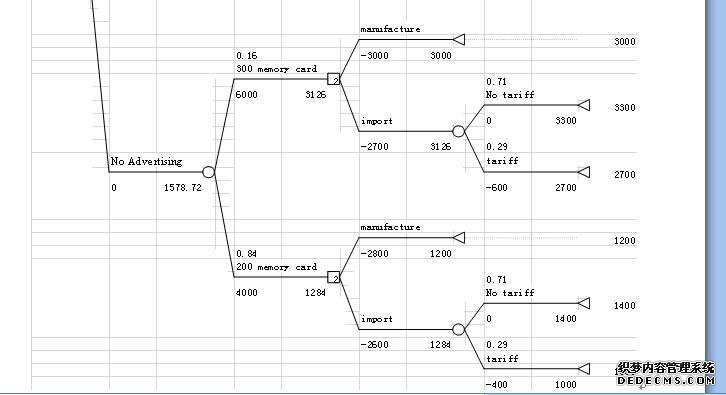# 管理学英国assignment解题范文

 Question 1.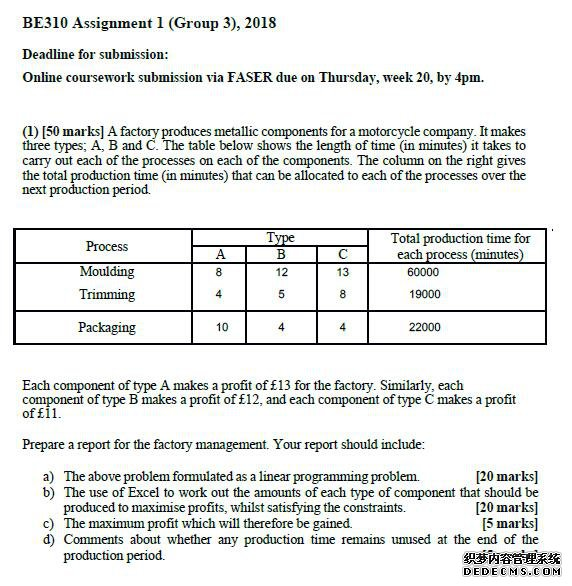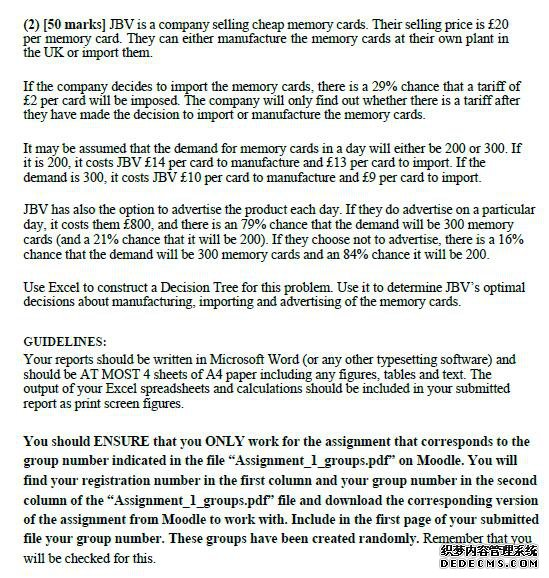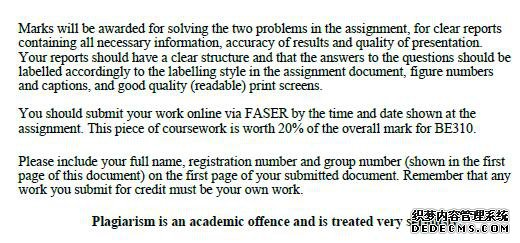Introduction介绍 摩托车公司生产金属部件有三种工艺，即成型、修整和包装。但是，每个过程的总生产时间都有限制。另一方面，有三种类型的金属部件：A、B和C。A型的每个部件为工厂赚取13英镑的利润。同样，B型的每个组成部分的利润为12英镑，C型的每个组成部分的利润为11英镑。本研究的目的是找到工厂的最大利润。 主体 线性规划模型 对于线性规划模型，本研究有以下决策变量。 x1是A型部件的数量； x2是B型部件的数量； x3是C型部件的数量； 本厂利润的目标函数最大值为：13*x_1+12%*x_2+11*x_3。 由于每种类型的组件都是独立的、不可分割的，所以对每种类型组件数量的约束是整数，并且等于或大于零。因此，寻找工厂最大利润的线性规划模型是 There are three processes on producing metallic components for a motorcycle company, that is, Moulding, Trimming and Packaging. However, there are limitation on the total production time for each process. On the other hand, there are three types of metallic components: A, B and C. Each component of type A makes a profit of £13 for the factory. Similarly, eachcomponent of type B makes a profit of £12, and each component of type C makes a profitof £11.The object of this study is to find the maximum profit for the factory. Main body The linear programming model For thelinear programming model, this research have the following decision variables.  X1 is the number of component of type A; X2 is the number of component of type B; X3 is the number of component of type C; The objective function of profit for the factory is Max: 13*X_1+12〖*X〗_2+11*X_3.  Since the components of each type are individual and inseparable, the constraints on the number of components of each type are integer and equal or larger than zero. Thus, thelinear programming model for finding the maximum profit for the factory is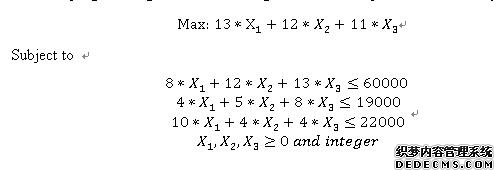Using Excel to work out the linear programming model  After establishing thelinear programming model for finding the maximum profit for the factory, this research will use the Excel Solver tool to work out the amounts of each type of components that should be produced to maximize profits, whilst satisfying the constraints. The specific results of the linear programming model are shown as follow.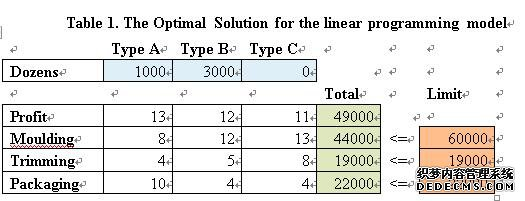The optimal solution for the component type A, B and C are 1000, 3000 and 0 respectively. In other words, the optimal number of component of type A, B and C are 1000, 3000 and 0 respectively. At these condition, the status on the constraint of Moulding is not binding. However, the status on the constraints of Trimming and Packaging are binding. Moreover, the maximum profit for the factory is £49000.  Maximum profit  Based on the optimal solution on the decision variables, the maximum profit for the factory is £49000.  Comments  The production time of Moulding remains unused at the end of the production period, and the production time of Trimming and Packaging does not remain unused. Even though the factory make full use of production time of Trimming and Packaging, there would remain the production time of Moulding. Thus, the production time of Moulding is more abundant than other process.    Question 2.  JBV is a company selling cheap memory cards. Their selling price is £20per memory card. They can either manufacture the memory cards at their own plant inthe UK or import them. In order to determine the optimal decision, there are many decision processes, advertising, the number of memory cards, manufacturing and importing of the memory. It is better to use a Decision Tree for this problem.   The first note of the decision tree is the decision note on advertising, if there is advertising, the cost is £800. If there is no advertising, the cost is £0.  The second note is the event note on the number of memory cards. If there is advertising, the probability of 300 memory cards is 79% and 200 memory cards is 21%. If there is no adverting, the probability of 300 memory cards is 16% and 200 memory cards is 84%.  The third note is the decision on manufacturing and importing. If the factory chooses to manufacture 300 memory cards, the cost is £10 per card. If the factory chooses to import 300 memory cards, the cost is £9 per card.If the factory chooses to manufacture 200 memory cards, the cost is £14 per card. If the factory chooses to import200 memory cards, the cost is £13 per card. The fourth note is theevent note on the tariff. The probability of importing memory cards with tariff is 29%, and the probability of importing memory cards with no tariff is 71%. In addition, the tariff is £2 per card.  This research will use the Excel to construct a Decision Tree to determine the optimal decisions.Based on the Decision Tree, the decision three shows that the optimal decisions are importing and advertising of the memory cards. Moreover, the expected profit on the decisions is £1939.18.